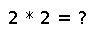# isapprox

isapprox(x, y; rtol::Real=sqrt(eps), atol::Real=0)

Inexact equality comparison: `true` if `norm(x-y) <= atol + rtol*max(norm(x), norm(y))`. The default `atol` is zero and the default `rtol` depends on the types of `x` and `y`.

For real or complex floating-point values, `rtol` defaults to `sqrt(eps(typeof(real(x-y))))`. This corresponds to requiring equality of about half of the significand digits. For other types, `rtol` defaults to zero.

`x` and `y` may also be arrays of numbers, in which case `norm` defaults to `vecnorm` but may be changed by passing a `norm::Function` keyword argument. (For numbers, `norm` is the same thing as `abs`.)

The binary operator `≈` is equivalent to `isapprox` with the default arguments, and `x ≉ y` is equivalent to `!isapprox(x,y)`.

## Examples

Checking you are not a robot: